# How to Calculate and Solve for Discharge | Slope Area Method | Water Budget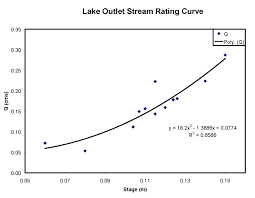The image above represents discharge | slope area method.

To compute for discharge | slope area method, four essential parameters are needed and these parameters are Manning’s Co-efficient (N), Area (A), Hydraulic Radius (R) and Slope (S).

The formula for calculating discharge | slope area method:

Q = (A / N) R2/3 S1/2

Where;

Q = Discharge
N = Manning’s Co-efficient
A = Area
S = Slope

Let’s solve an example;
Find the discharge when the manning’s co-efficient is 4, the area is 30, the hydraulic radius is 15 and the slope is 22.

This implies that;

N = Manning’s Co-efficient = 4
A = Area = 30
R = Hydraulic Radius = 15
S = Slope = 22

Q = (A / N) R2/3 S1/2
Q = (30 / 4) (15)2/3 (22)1/2
Q = (7.5) (6.08) (4.69)
Q = 213.96

Therefore, the discharge is 213.96.

Nickzom Calculator – The Calculator Encyclopedia is capable of calculating the discharge | slope area method.

To get the answer and workings of the discharge | slope area method using the Nickzom Calculator – The Calculator Encyclopedia. First, you need to obtain the app.

You can get this app via any of these means:

To get access to the professional version via web, you need to register and subscribe for NGN 1,500 per annum to have utter access to all functionalities.
You can also try the demo version via https://www.nickzom.org/calculator

Apple (Paid) – https://itunes.apple.com/us/app/nickzom-calculator/id1331162702?mt=8
Once, you have obtained the calculator encyclopedia app, proceed to the Calculator Map, then click on Agricultural under Engineering.Now, Click on Water Budget under AgriculturalNow, Click on Discharge| Slope Area Method under Water Budget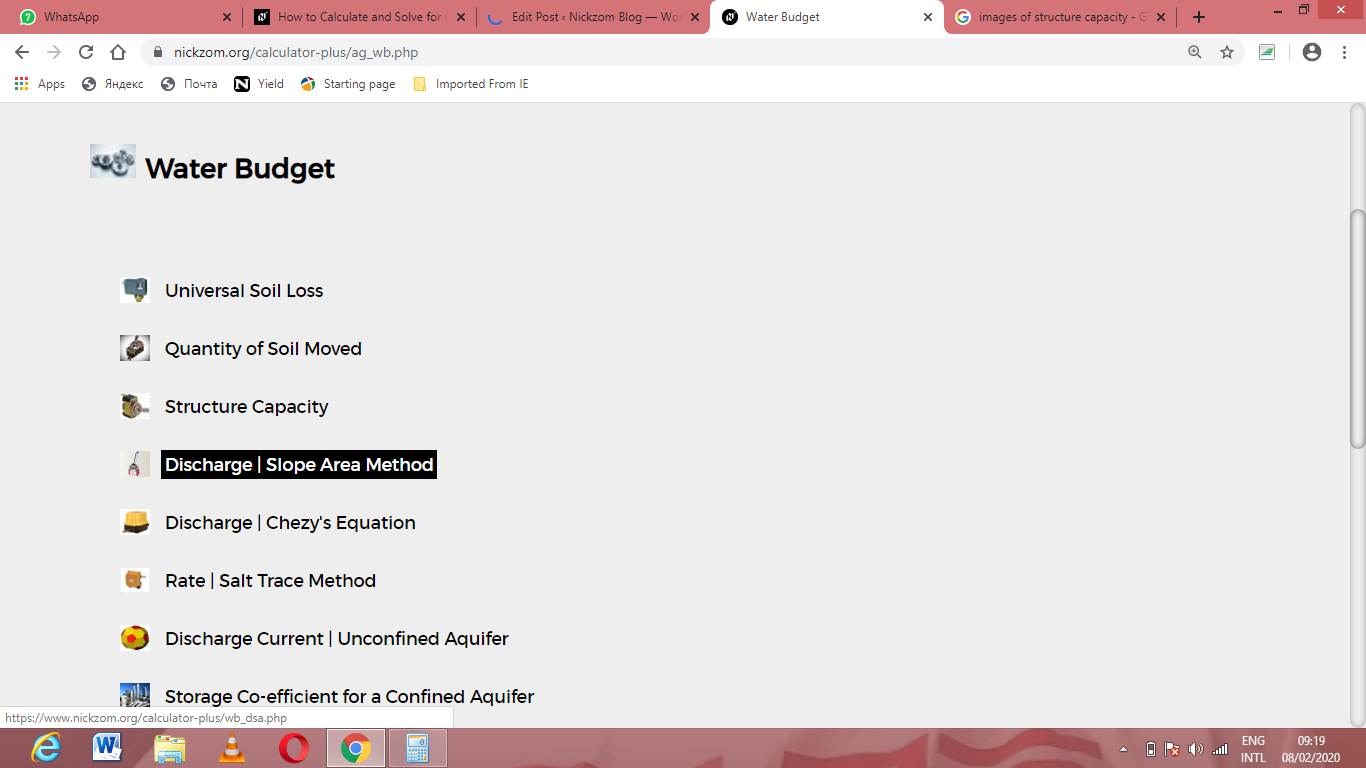The screenshot below displays the page or activity to enter your values, to get the answer for the discharge | slope area method according to the respective parameters which are the Manning’s Co-efficient (N), Area (A), Hydraulic Radius (R) and Slope (S).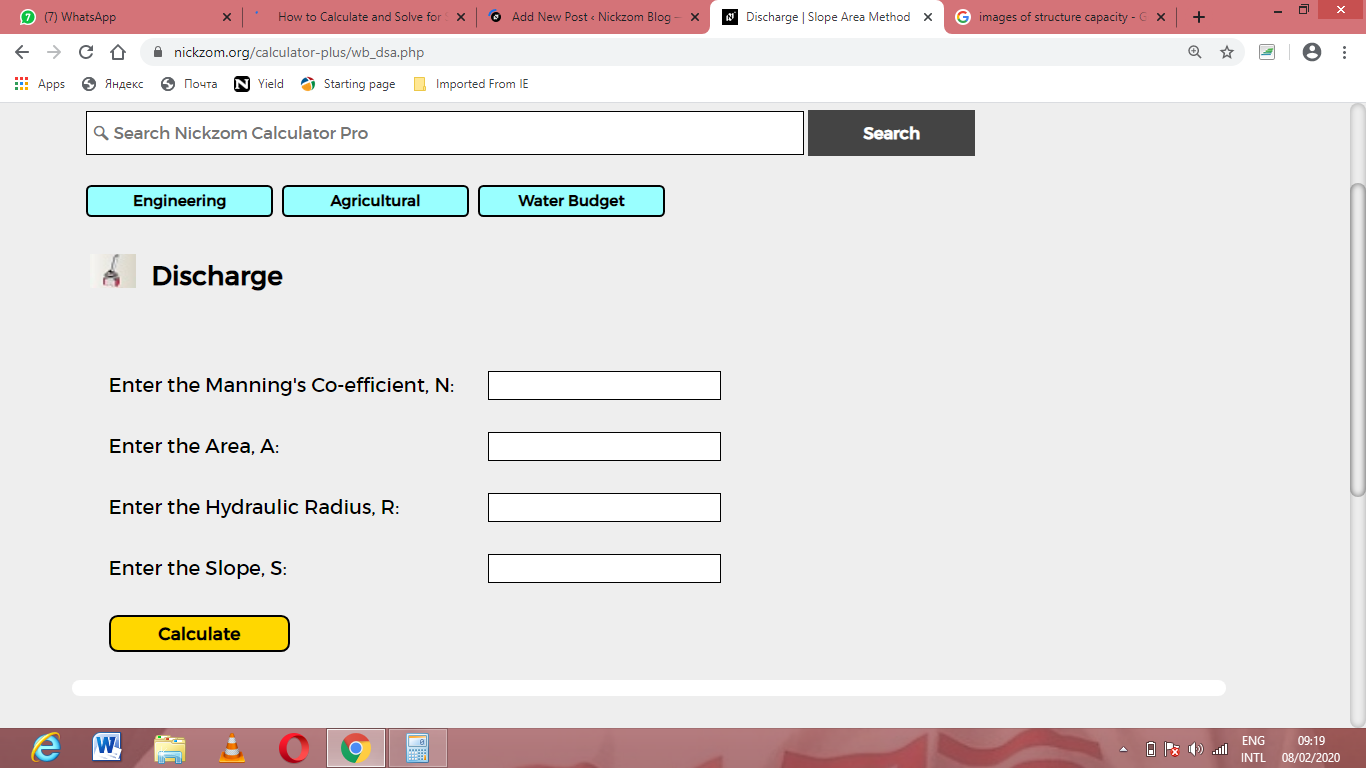Now, enter the values appropriately and accordingly for the parameters as required by the Manning’s Co-efficient (N) is 4, Area (A) is 30, Hydraulic Radius (R) is 15 and Slope (S) is 22.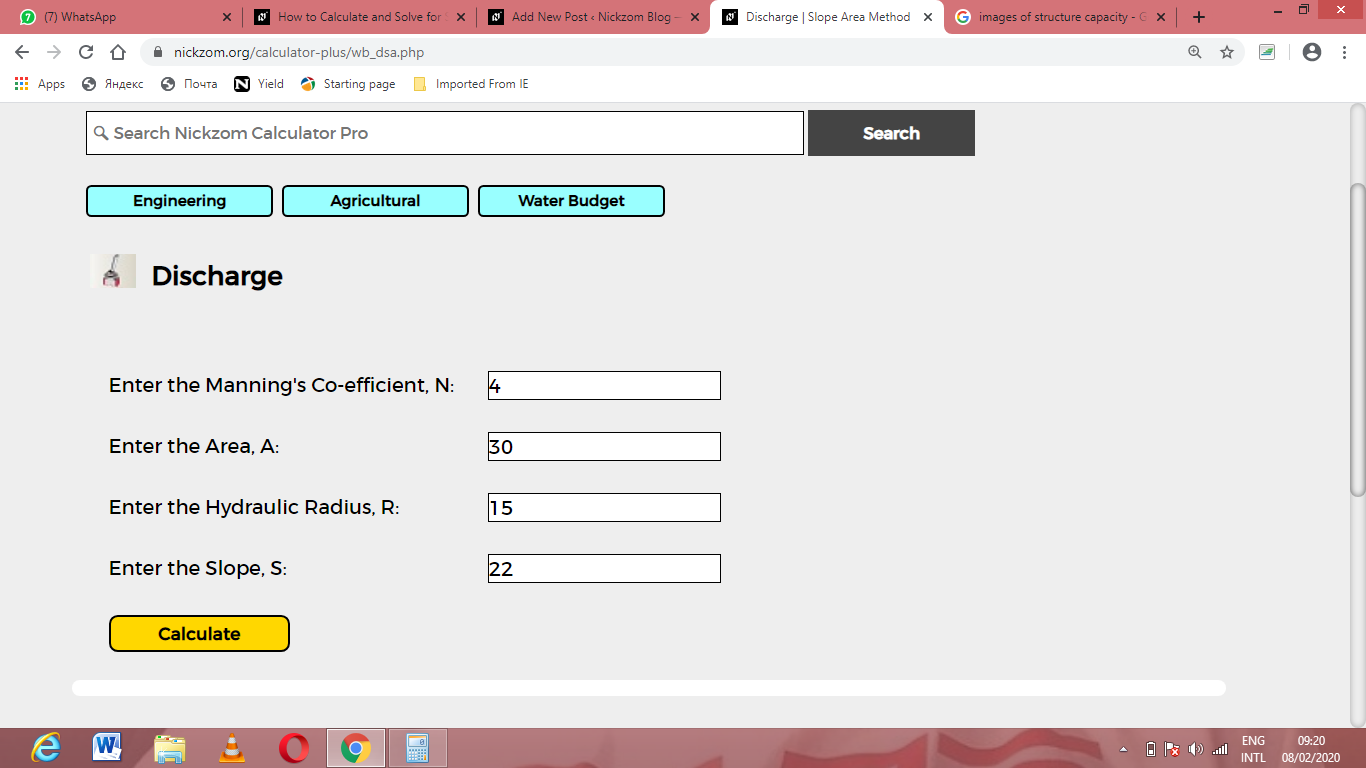Finally, Click on Calculate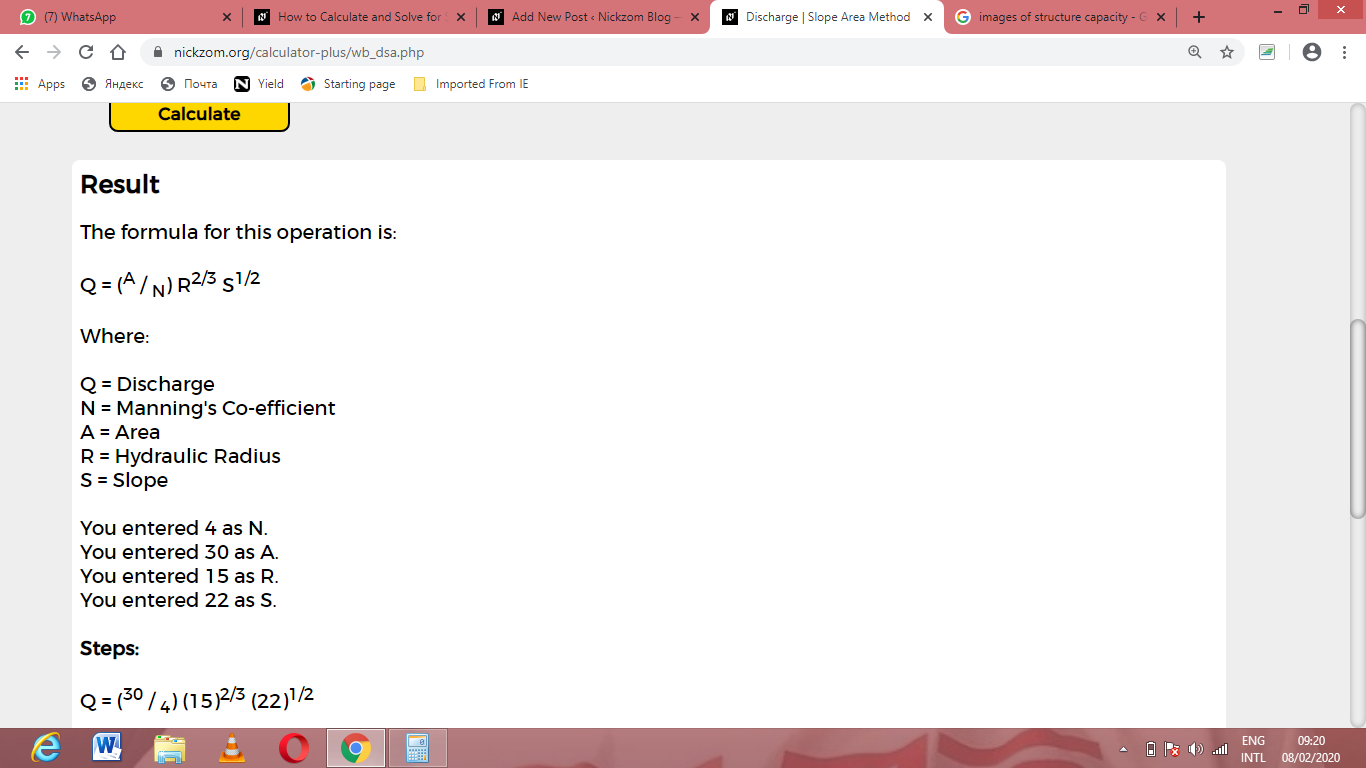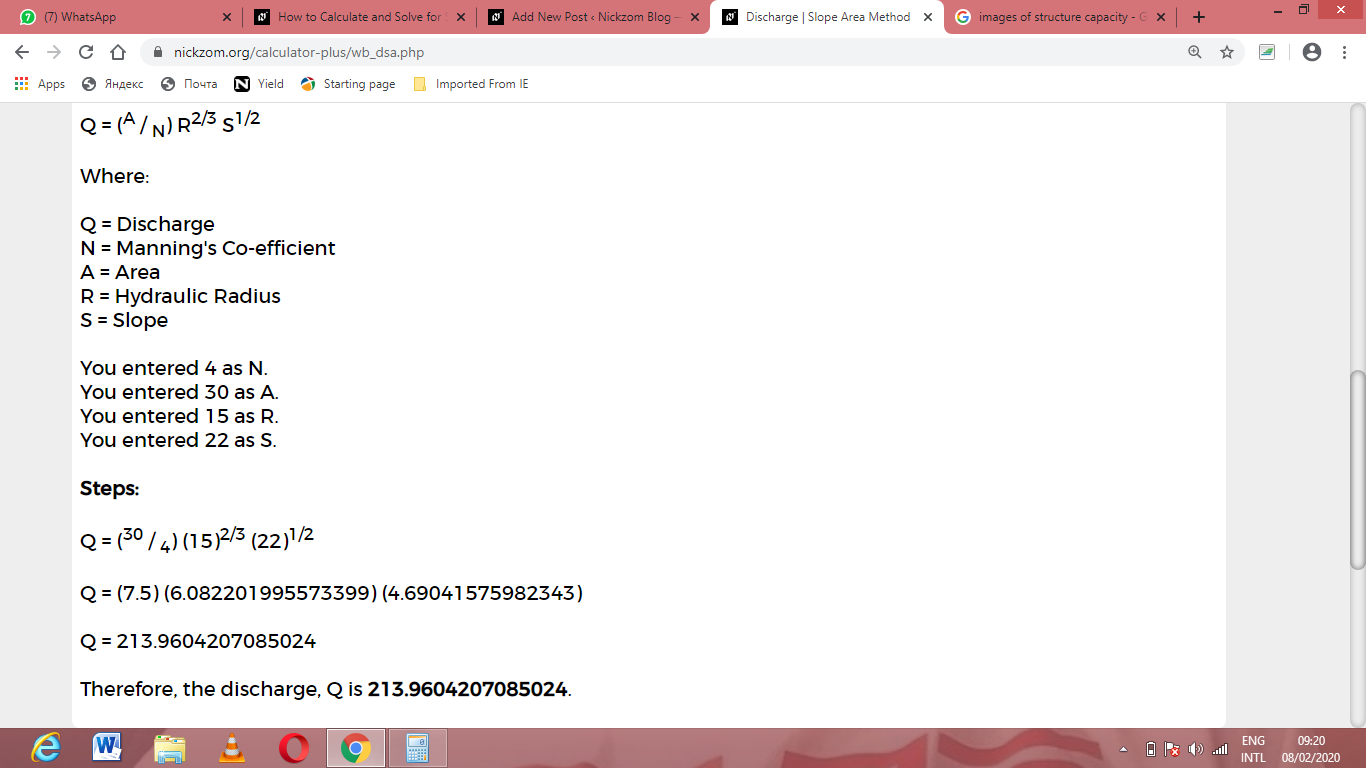As you can see from the screenshot above, Nickzom Calculator– The Calculator Encyclopedia solves for the discharge | slope area method and presents the formula, workings and steps too.